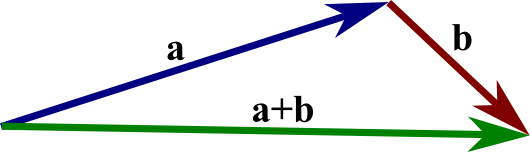# Math Insight

### Image: The sum of two vectorsGiven two vectors $\vc{a}$ and $\vc{b}$, we form their sum, $\vc{a}+\vc{b}$, by translating the vector $\vc{b}$ until its tail coincides with the head of $\vc{a}$. Then, the directed line segment from the tail of $\vc{a}$ to the head of $\vc{b}$ is the vector $\vc{a}+\vc{b}$.

Image file: vector_a_plus_b.png

Source image file: vector_a_plus_b.svg
Source image type: Inkscape SVG